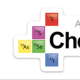# Resistance is not futile when it comes to buffersDecember 31, 2021

I quite often hear people say words to the effect of, “A buffer is a solution which does not change its pH when a strong acid or base is added to it.” Of course, this is not true, and instead I use the phrase, “A buffer is a solution that resists change in its pH when a strong acid or base is added to it.” This is a subtle, but important difference.

Here’s a little calculation** that you can use to neatly illustrate the point, even though this would not be asked on the exam according to the exclusion statement in 8.9 that says, COMPUTATION OF THE CHANGE IN pH RESULTING FROM THE ADDITION OF AN ACID OR A BASE TO A BUFFER WILL NOT BE ASSESSED ON THE AP EXAM.

Consider two scenarios;

a. Firstly, 1.0 mL of 2.0 M HCl being added to 100 mL of pure water at 298 K.

b. Secondly, 1.0 mL of 2.0 M HCl being added to 100 mL of a buffer solution that has 0.10 M ethanoic acid, and 0.15 M sodium ethanoate. (Ethanoic acid has a Ka = 1.8 x 10-5)

In the first case, the original [H3O+]  = 1.0 x 10-7 M, so the pH = – log(1.0 x 10-7) = 7.00

Addition of the HCl adds 0.002 moles of H+, gives a total volume of 101 mL, and consequently changes the concentration of H3O+ to (0.0020001/0.101) = 0.0198029703. The result is that the pH = -log(0.0198029703) = 1.70

The addition of the acid caused the pH to fall from 7.00 to 1.70. The pH changed by 76% in terms of the pH scale*.

In the second case, the pH of the original buffer solution can be calculated using the Henderson-Hasselbalch equation, pH = -log(1.8 x 10-5) + log(0.15/0.10) = 4.92

Addition of the HCl adds 0.002 moles of H+, which reacts with the base present in the buffer, to create the conjugate acid in the following reaction; CH3COO + H+ → CH3COOH, reducing the number of moles of base from the original 0.015 to 0.013, and increasing the moles of acid from the original 0.010 to 0.012.

Re-applying the Henderson-Hasselbalch equation, pH = -log(1.8 x 10-5) + log(0.013/0.012) = 4.78

The addition of the acid caused the pH to fall from 4.92 to 4.78. The pH changed by 2.8% in terms of the pH scale*.

*This seems like a problematic stat to me, BUT it serves our purpose here, and makes the point about buffers resisting a change in pH (but not having static pHs), rather nicely.

The other thing I like say in order to ‘explain’ the small change in pH in a buffer situation like the one outlined above, is to say that the reaction between the conjugate base and the strong acid, i.e.,  (CH3COO + H+ → CH3COOH), converts a strong acid (H+) into a weak acid, (CH3COOH), so its effect is less. Again, this is a huge oversimplification, but I kind of like the comfort that it brings.

**Some calculations inspired by notes on buffers by Lew Acampora

1.Love the explanation that “the reaction between the conjugate base and the strong acid, i.e., (CH3COO– + H+ → CH3COOH), converts a strong acid (H+) into a weak acid, (CH3COOH), so its effect is less.”

•Yeah, it kinda helps (I think).